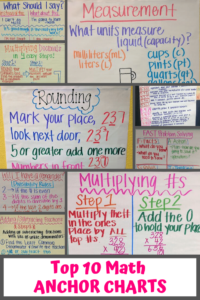# 10 Best Math Anchor Charts for Elementary School Classrooms

Math anchor charts are one of the most powerful teaching strategies in my classroom.  My goal with an anchor chart is to help students understand, visualize, and memorize how to solve math problems and understand math concepts.

I wholeheartedly believe that anchor charts work.  Time and time again during tests, I see students looking towards the walls where my anchor charts would hang when trying to answer math questions.

As such, I want to share with you some of my top math anchor charts.

## What is a math anchor chart?

This is an important question you may have if you’ve never heard of anchor charts before.   A math anchor chart helps to “anchor” students’ learning when tackling new concepts.  Created together with teacher and student, the anchor chart is a visual reminder for students that covers both the topic and relevant problem-solving strategies.   I find that having anchor charts supports my teaching and help students organize their thoughts better.

For example, a rounding anchor chart will remind students of place value and what rounding is and then may display some important strategies to use when rounding numbers.

## How To Make An Anchor Chart With Your Students

I’ve found the best way to solidify a math concept for students is to create the anchor chart with them during class.  If they’re engaged in the process, they will retain the information better.

If you’re a perfectionist like I am, you can create an anchor chart with your students first.  Then, when they’ve gone home for the day, you can make a prettier one all on your own to hang on the wall.

## My Best Math Anchor Charts

Here are some of my favorite anchor charts over the years.   (Since I have taught both third and fifth-grade math, there are some third-grade math anchor charts and some fifth-grade math anchor charts.)

### 1. Multiplying Decimals Anchor Chart

There are four easy steps:

1. Round your numbers and find the product for a reasonable estimate.
2. Multiply your numbers without the decimal point.
4. Double-check the placement of your decimal point.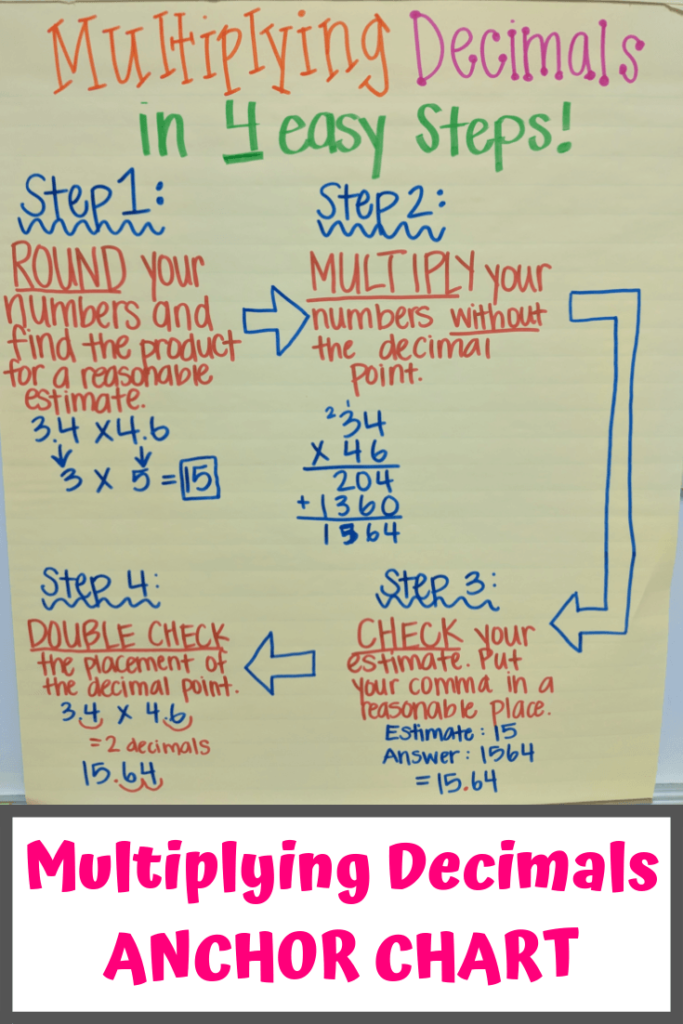This decimal multiplication anchor chart has helped my students tremendously. My students kept jumping ahead by multiplying their numbers and then randomly placing the decimal. This helps them to slow down a little and actually understand the concept of decimal multiplication.

### 2. Adding and Subtracting Fractions Anchor Chart

Steps for adding and subtraction fractions with like or unlike denominators:

1. Find the least common denominator and rewrite the fractions.
2. Add or subtract the numerators.
3. Leave the denominator the same.
4. Simplify if possible.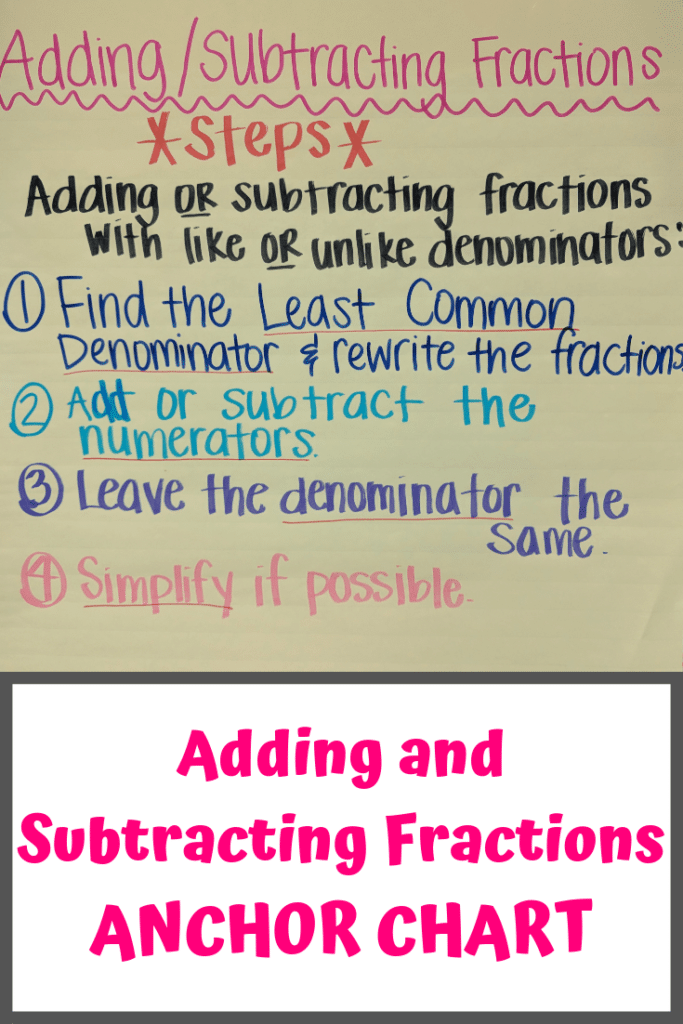These steps on my fractions anchor chart allowed my students to master adding and subtracting fractions easily. Definitely a great go-to when reinforcing those few basic skills.

### 3.  Rounding Decimals Anchor Chart-5th Grade

This rounding anchor chart for fifth graders teaches the students to:

• Mark your place, look next door.
• 5 or greater, add one more.
• The numbers in the front stay the same.
• Numbers behind zeroes their name.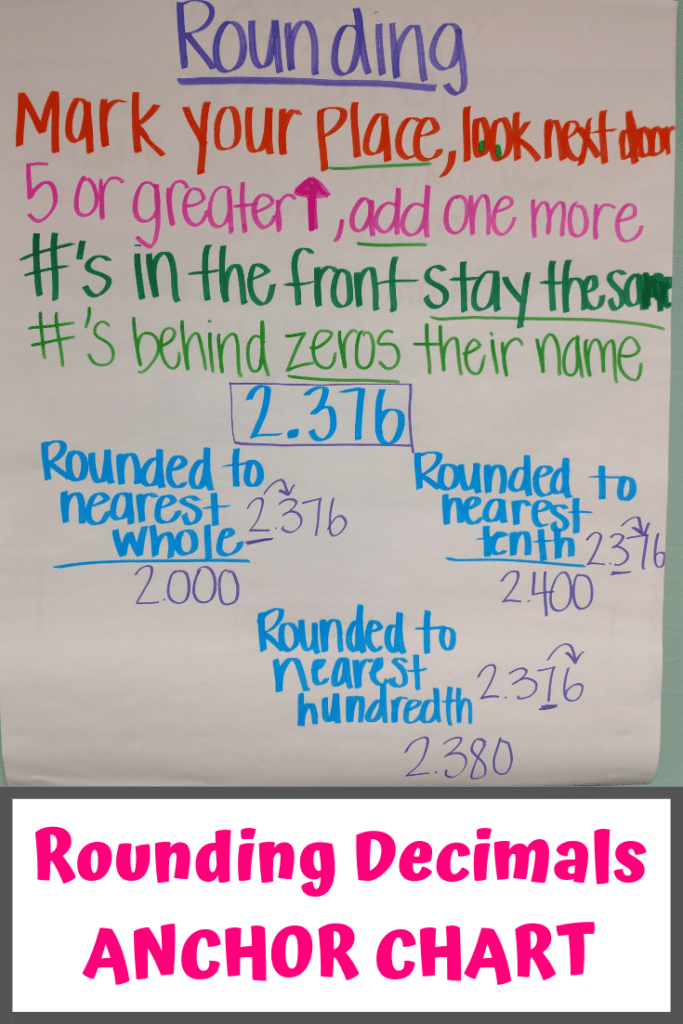This anchor chart is almost the same chart I used for my third graders. Remembering these little rhymes helps them to remember the process of rounding. In addition to rounding, I like to refer back to this for place value.

### 4. Rounding Whole Numbers Anchor Chart- 3rd Grade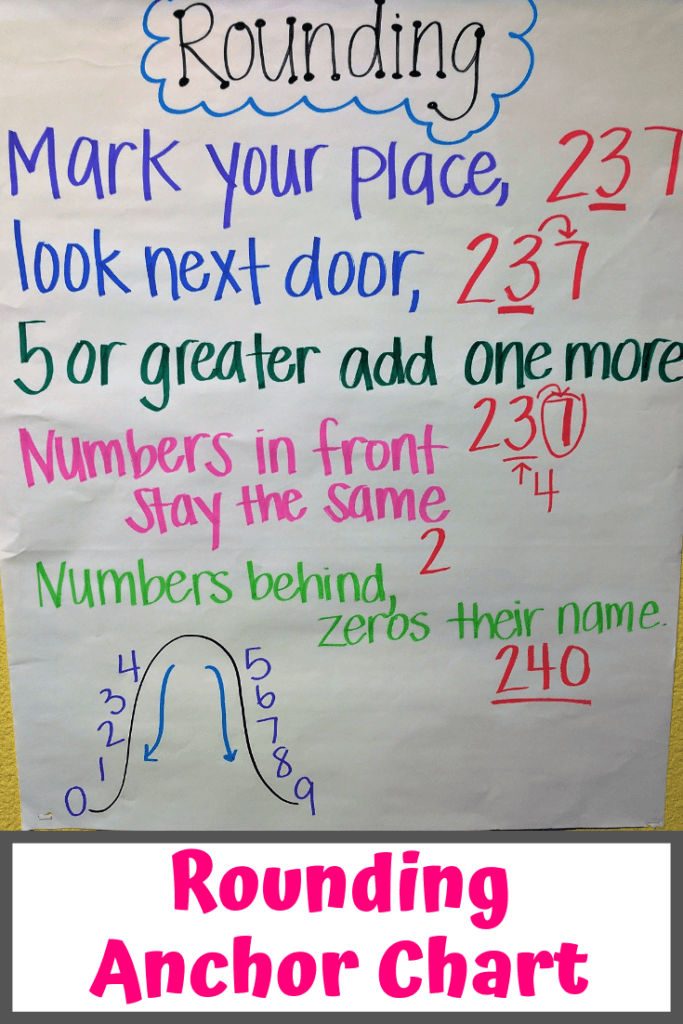Very similar to the above fifth-grade math anchor chart, here’s my third-grade math rounding anchor chart.  When teaching rounding to third graders, you can never go wrong with a visual. The good-ole roller coaster analogy is the best.

### 5. Multi-Digit Multiplication Anchor Chart: Multiplying 3 digits by 2 digits

Here are the steps I recommend on this multiplication anchor chart:

• Multiply the number in the ones place by all top numbers.
• Multiply the tens place by all top numbers.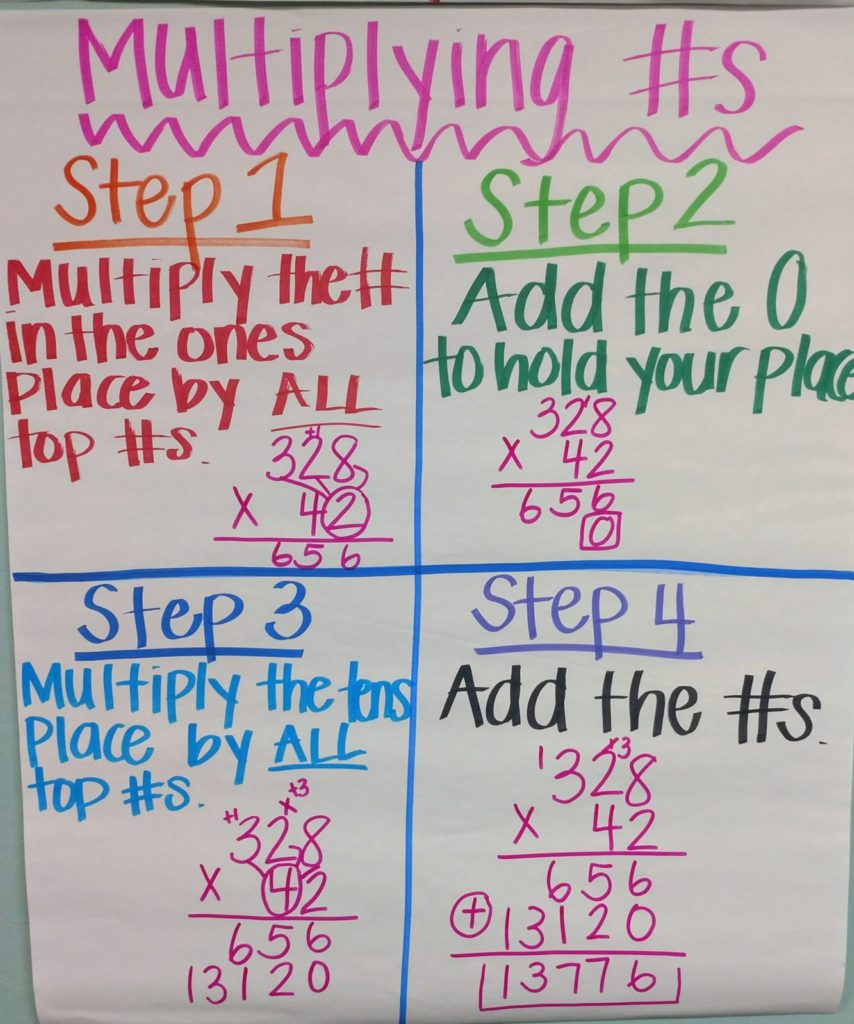At the beginning of the year, my students struggled with multiplying. Once again, creating a list of steps helped them the most. By having this hung up all year, they were able to refer back to it. By the time the STAAR test came around, they had all of the steps memorized.

### 6. Divisibility Rules Anchor Chart: Will I Have A Remainder?

Here are some of these divisibility rules on this math anchor chart.

• 2: if the number is even
• 3: if the sum of the digits is divisible by 3
• 4: if the last 2 digits are divisible by 4
• 5: if the last digit is a 0 or 5
• 6: if the number is divisible by 2 and 3
• 9: if the sum of the digits is divisible by 9
• 10: if the last digit is a 0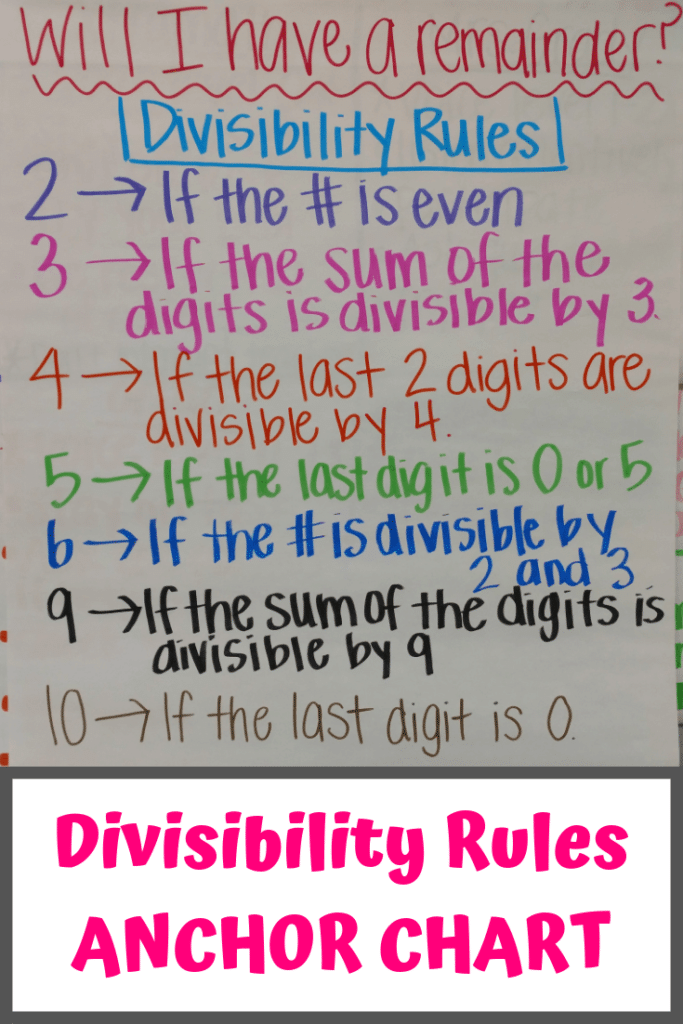This is always great to have around. Believe it or not, I use these divisibility rules daily. If you can get your kids to remember these rules, division could be a lot easier for them.

### 7. FAST Problem Solving Chart

The acronym FAST stands for facts, action, solve, and thinking.

####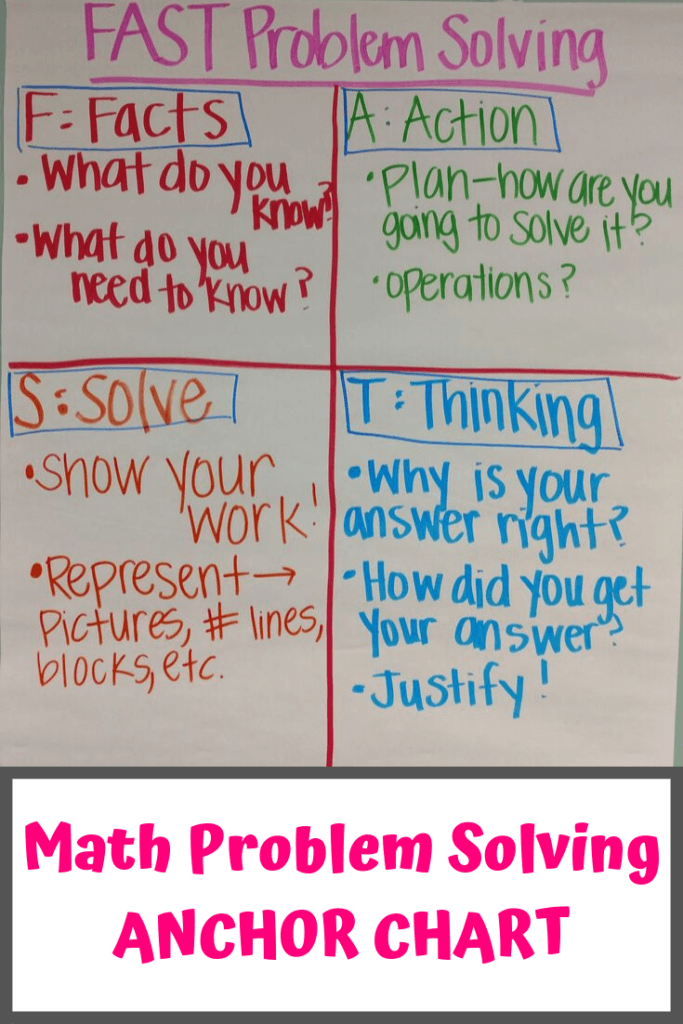FAST problem solving can be a great way to get your students writing in the classroom. I don’t necessarily think this anchor chart is showing the best strategy for your kids to solve every problem. However, for those struggling learners, this is a great way for them to get the process of problem-solving going.

### 8. Units of Measurement Anchor Chart

This measurement math anchor chart asks two questions.

1. What units measure liquid (capacity)?
2. What units measure weight (mass)?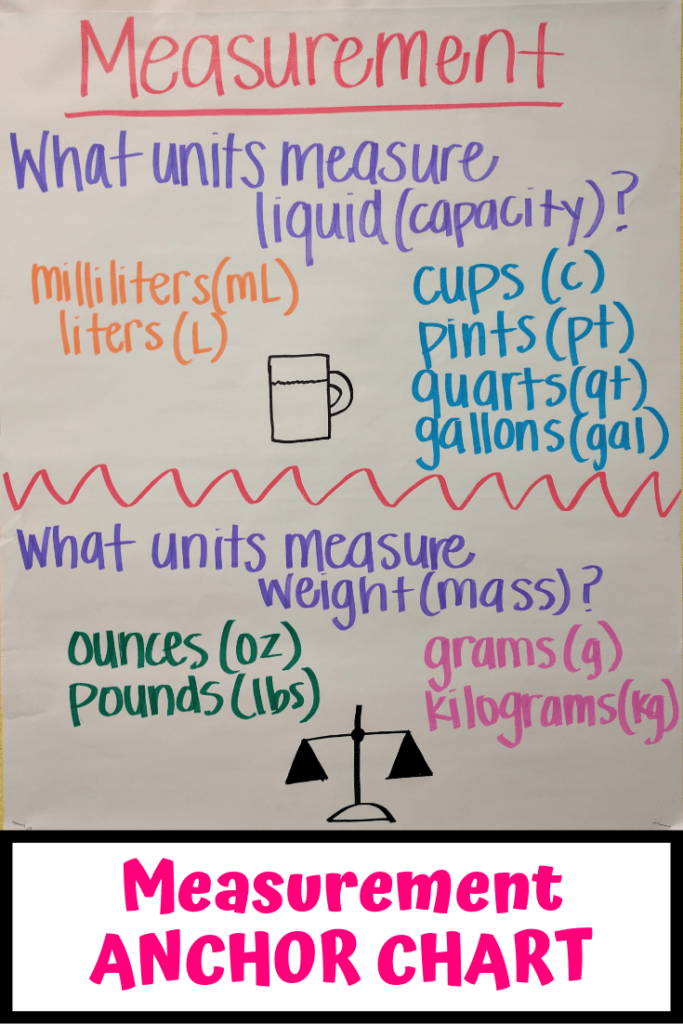My students always struggled the most with remembering what each unit measured. This anchor chart is a great way for the kids to associate which units measure capacity and which ones measure weight.

### 9. TEKS Objectives Displayed

####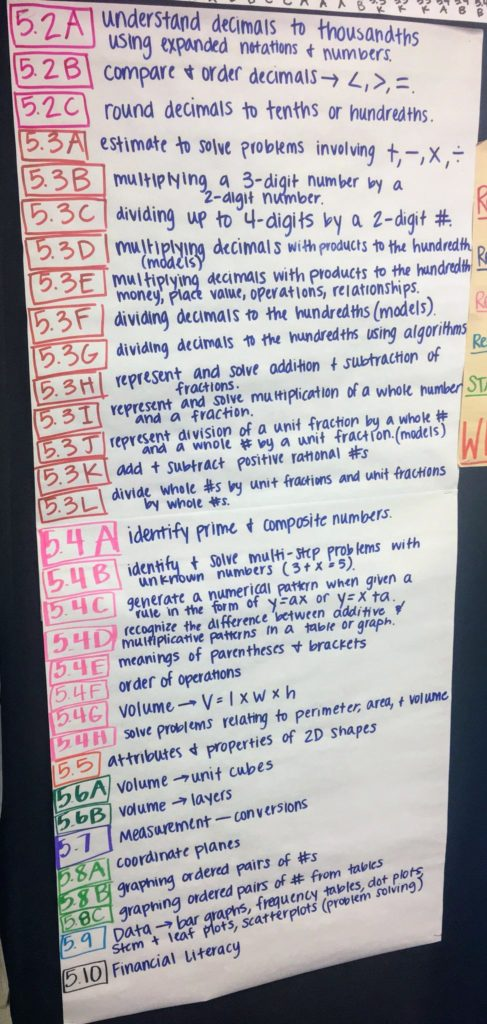Holding my students accountable has become a breeze by having this TEKS anchor chart in my classroom.  (TEKS are our learning standards here in Texas.) The students can see exactly what they are responsible for learning and knowing. We refer to these every day throughout our lessons and our learning targets.

I have this as a part of my classroom data wall.

### 10. Proactive Language to Motivate Math Students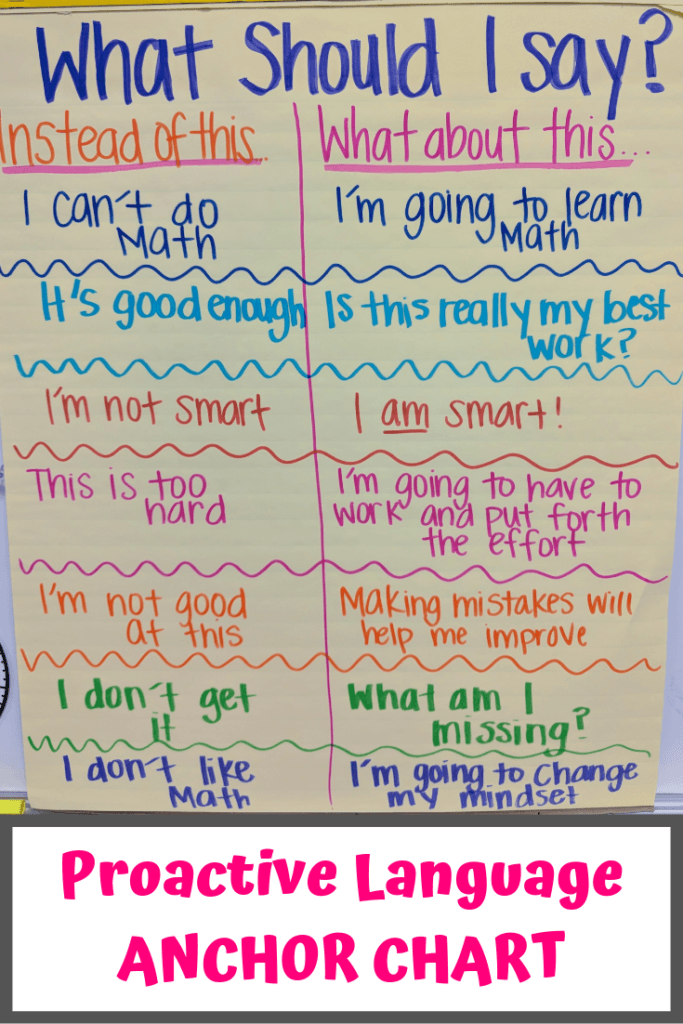This proactive language anchor chart is by far my favorite anchor chart I have ever created. I was to the point where I was so frustrated with the language my students were using in the classroom. Once I began the talk of proactive language, not only did my students’ attitude change, but their test scores changed as well.

## Honorable Mention Math Anchor Charts

Two anchor charts didn’t make it into my top 10, but I still think they’re worth sharing.

### Area and Perimeter Anchor Chart

This isn’t one of my most inventive anchor charts, so I’m not including it in my top 10 math anchor charts.  However, I do think this area and perimeter anchor chart can help certain students who are struggling to understand the concept.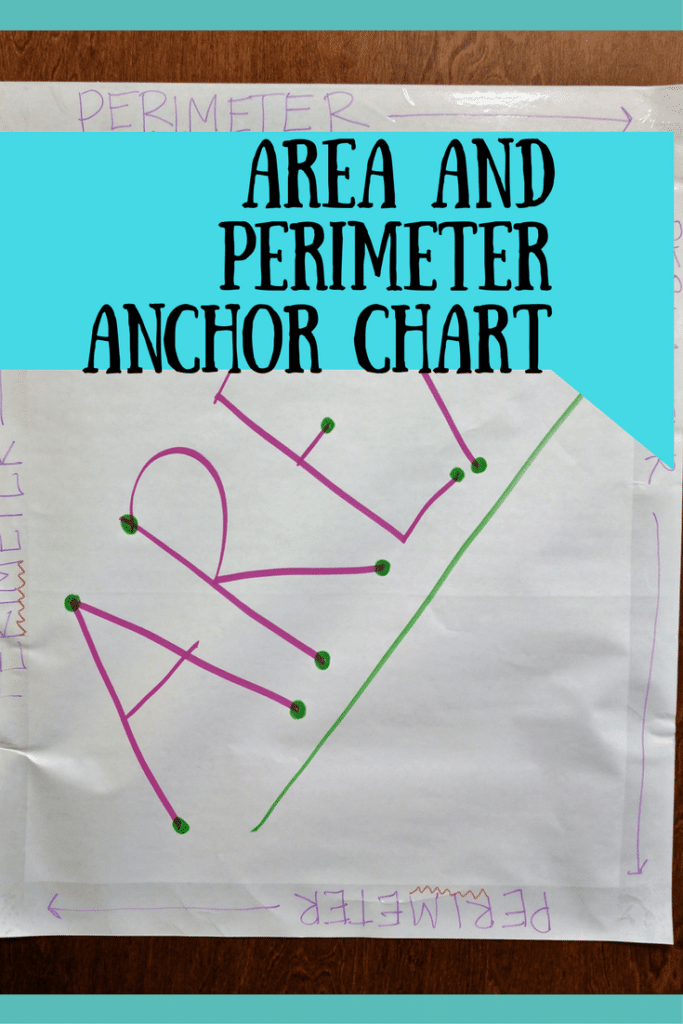### Classroom Rules – “10 Rules of Math” Anchor Chart

Since this isn’t as much an anchor chart as a clever display of my classroom rules, it doesn’t make the top 10. However, having classroom rules is important in any classroom, math or not.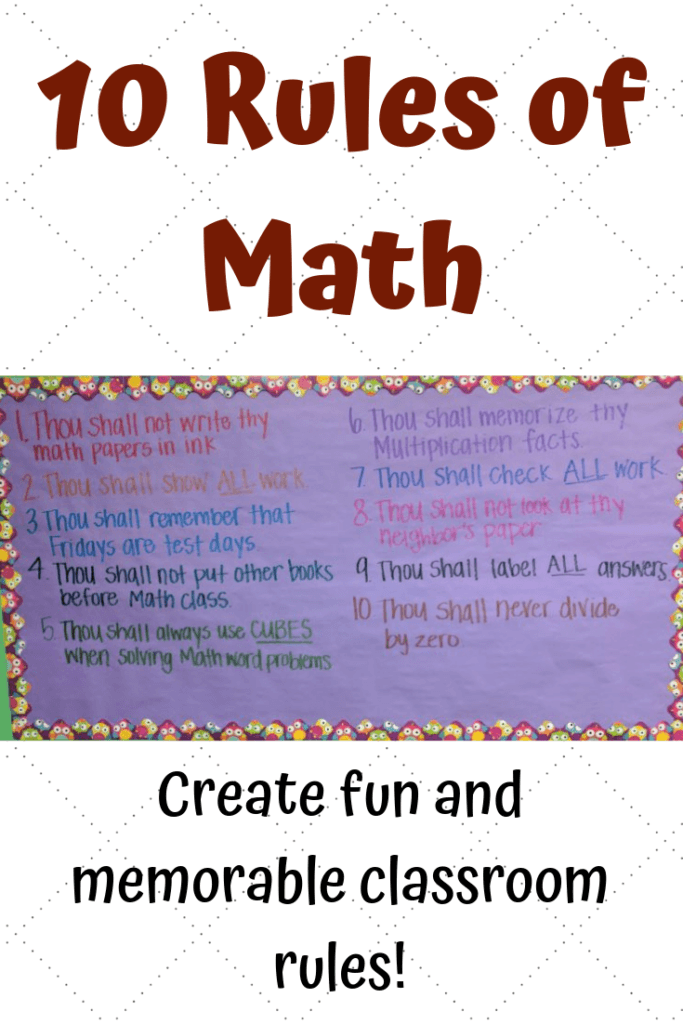And that’s it!  What other math anchor charts do you like in your elementary school classroom?

Check out other teaching ideas while you’re here!